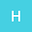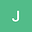Low Computational Complexity Methods for Decoding of STBC in the Uplink of a Massive MIMO Systems
••• hosein,
• j.pourrostam
hosein
University of Tabriz
Author Profilej.pourrostam
University of Tabriz
Author Profile## Abstract

Reducing computational complexity of the modern wireless communication systems such as massive Multiple-Input Multiple-Output (MIMO) configurations is of utmost interest. In this manuscript, we propose new algorithm that can be used to accelerate matrix inversion in the decoding of space-time block codes (STBC) in the uplink of dynamic massive MIMO systems in different scenarios. A multi-user system in which the base station is equipped with a large number of antennas and each user has two antennas is considered. In addition, users can enter or exit the system dynamically. For a given space-time block coding/decoding scheme the computational complexity of the receiver will be significantly reduced by employing the proposed methods. First scenario is utilizing an algorithm that approximates inverse matrix in linear decoding by employing Neumann series. Also, second scenario is using an algorithm to reduce the computational complexity of decoder when the user is added to or removed from the system. In the proposed scheme, the matrix inversion for zero-forcing (ZF) and minimum mean square error (MMSE) decoding is derived from the inverse of a partitioned matrix and the Woodbury matrix identity. Furthermore, the suggested technique can be utilized when the number of users is fixed but the channel state information (CSI) changes for a particular user. The mathematical equations for both scenarios are derived and their complexity are compared to the direct way of computing the inverse. Also, their performance is evaluated in terms of bit error rate. Evaluations confirm the effectiveness of the proposed approaches.